What is a Resistor, and how to choose the correct resistor for your circuit?

In a conductor or a semiconductor, Resistance is the property of opposing the flow of electrons. The electronic components having resistance against the flow of electrons in a circuit is known as a resistor. You must have seen these resistors soldered on printed circuit boards in your electronic gadgets.
Resistance is measured in ohms, symbolised by the Greek letter omega (Ω). Ohm SI unit of resistance is named after Georg Simon Ohm (1784-1854), a German physicist who studied the relationship between Voltage, Current and Resistance.
“Ohm’s law states that the voltage across a conductor is directly proportional to the current flowing through it, provided all physical conditions and temperature remain constant.”

R = V / I
R: Resistance
V: Voltage
I: Current

To some extent, all materials resist current flow. Based on their resistivity, materials are classified into two categories.

Conductors: Materials with low resistance that allow electrons to travel freely. For example, Silver, copper, gold, and aluminium.

Insulators: Materials with high resistance that prevent electrons from flowing freely. For example, Rubber, paper, glass, wood, and plastic are some examples.

The symbol for a constant resistor is as shown below.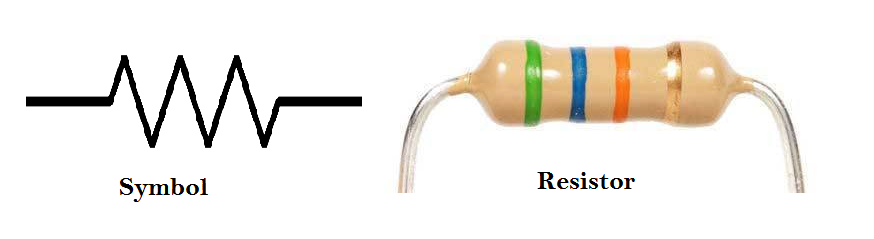Various types of resistors are available, but the most common ones are carbon film resistors (carbon resistor) and metal oxide film resistors.
These are also known as Through-Hole Resistor or Radial Axis Resistors.How to read resistor color code?The Colour Coding method is used to determine resistors’ value, and each resistor has its own resistor color code. Generally, 4 color bands are used to identify the exact value of resistors, as shown below. Each colour has some significant digit within a range of 0 to 9. The resistor color coding formula is very simple.

If you want to know the 10k ohm resistor color code, just look at the table below and get the correct colour code, i.e. Brown, Black and Orange.

Resistor Color Code Chart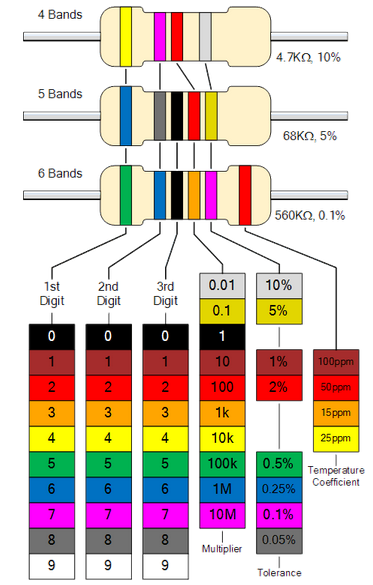The first and second digits of the value are represented by the first and second coloured bands, respectively, while the third colour band shows the multiplier number of zeroes added. The fourth colour band indicates the tolerance value.
The range of values that a resistor may withstand without being destroyed is referred to as tolerance. This is a crucial consideration. The color coding determines the value of a resistor as shown in the diagram below.

5-Band Resistor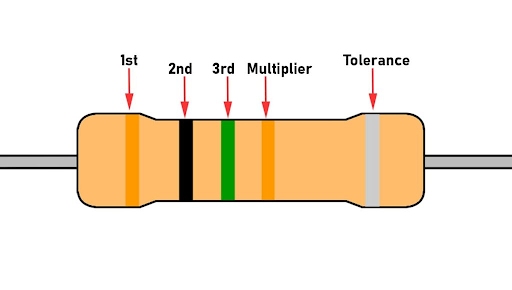The five-colour band resistors are manufactured with a tolerance of 2% and 1%. You can use these resistors with high precision circuit. 5 band resistor color code gives the value of resistor where the first three bands represent digits, the fourth one indicates multiplier, and the fifth represents tolerance.

6 Band Resistor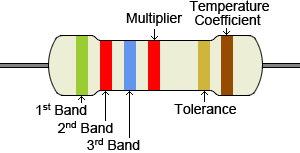The six-colour band resistors are manufactured with a tolerance of 2% and 1% and also for other high accuracy resistors. In these five band resistors, the first three bands represent digits, the fourth one indicates multiplier, the fifth represents tolerance, and the sixth band represents temperature coefficient.

3 Band ResistorThe 3-band resistor has three colours with a multiplier and no tolerance band. The resistors colour give you the exact value of the resistor with 20% Tolerance..

Many resistor color code calculators are available online to determine the resistance value but it is always better to remember the color scheme used with resistors.
There is no difference between carbon resistor color code and metal film resistor color code.

Common Resistor Values in Ohm 4 band Resistors (20 tolerance)
1M Resistor Color Code Brown, Black, Green
1 ohm Resistor Color Code Brown, Black, Gold
10k resistor Color code Brown, Black, Orange
1k Resistor Color Code Brown, Black, Red

When a resistor is used in a circuit, it can be connected in series, parallel, or both. Let us see what happens to the total current, voltage, and resistance value when they are connected in series as well as in parallel.

Resistors in SeriesResistors connected in series will give us the total resistance value equal to the sum of all individual resistances.
The total resistance value of the resistor network shown above is

R=R1+R2+R3
R= 1kΩ+5kΩ+9kΩ
R= 15kΩ

Similarly, the total voltage that appears across a series resistors network is the addition of voltage drops at each individual resistance.

V=V1+V2+V3
And, the total amount of current that flows through a set of resistors connected in series remains the same at all the points throughout the resistor network.
I=I1+I2+I3

Resistors in Parallel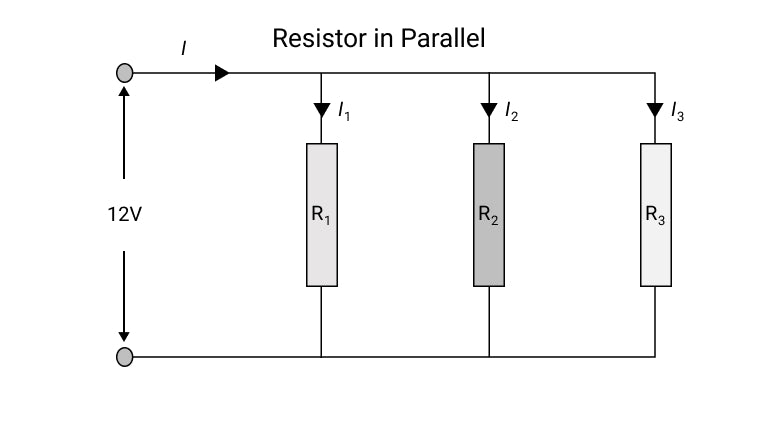To calculate the total resistance value of resistors connected in parallel, Individual resistances' reciprocal 1/R values are added with the inverse of the algebraic sum to get the total resistance value.
The total resistance value of a resistor network shown above is

1/R=1/R1+1/R2+1/R3

The total voltage that appears across a series resistors network is same as the voltage drops at each resistance.

V=V1+V2+V3

And, the total amount of current entering a Parallel resistive network is the sum of all individual currents flowing in all Parallel branches.

I=I1+I2+I3

There are various types of resistors depending upon their properties and applications.Non-linear resistors show non-linear VI characteristics, while linear resistors show linear VI characteristics. Other elements such as temperature and light affect the voltage and current values, however, they are not always linear.

Difference between carbon film and wire-wound resistors
Carbon Film Resistor

These resistors are made of carbon or graphite material. Generally comes in small size and are lightweight. Carbon film resistors are suitable for high-frequency applications. They also have a low-temperature coefficient value.
It is very difficult to show linear behaviour during changes in temperature, humidity, etc. The current carrying capacity of these resistors is also limited to about 2 Watts. While manufacturing, it is difficult to make a resistor with a value < 2 ohms.

Wire Wound Resistor

These resistors are made by winding a wire on an insulating material.
Wire wound resistors have a high power-rating with a low tolerance value. It is easy to make resistors of lower values (0.01 ohm), unlike carbon film resistors and also comes with a higher order of stability and reliability.
But, It is difficult to manufacturer wire-wound resistors of higher value in Mega ohms. These resistors are also not very suitable for high-frequency applications. Usually, they come in size bigger than carbon film resistors, making them unsuitable for various small size circuits.

SMD Resistor

Chip surface-mount resistors are smaller than through-hole resistors, making them ideal for printed circuit boards (PCBs). Pull-up/pull-down operations to guarantee a signal is at a proper logic level when external devices are removed, voltage divisions, current limiting, and filtering signals at certain frequencies in high-pass/low-pass/band-pass filters are some of their popular applications. To disable a piece of a circuit, you can use 0 resistors as jumpers.

Chip SMD Resistors come in two varieties:

1. Thin-film resistors are utilised in high-precision applications such as audio, medical, and test equipment. Compared to thick film resistors, they have less fluctuation (0.1% to 2%), a lower temperature coefficient (5 ppm/K), and less noise.
2. Thick Film resistors are the most popular and widely used form of the resistor. They have a larger temperature coefficient (50 ppm/K) and are noisier than thin-film resistors, with a fluctuation of 1% to 5%. Thick film resistors are usually the first choice if there are no special performance requirements.

Package: 0201, 0402, 0603, 0805, and 1206 packages are the most common. The numbers represent the dimensions in the imperial system, with 0402 being 0.04 X 0.02 inches and 0603 being 0.06 X 0.03 inches and so on.

Variable ResistorsVariable resistors are ones whose values may be changed manually to meet the needs of the application. With the help of a shaft attached, a specific resistance value is selected from a range of resistance values. A variable resistor is represented by the symbol below.Significance of Power rating when choosing a resistor for your circuit?

Power Rating
If the current flow increases while using a resistor, the resistor generates heat. The resistor may get damaged if this value exceeds a particular critical value. In order to avoid this, the wattage rating of a resistor should be chosen carefully. In simple words, power rating decides the tolerance of the resistor against the value of current flowing through it.

Passive components